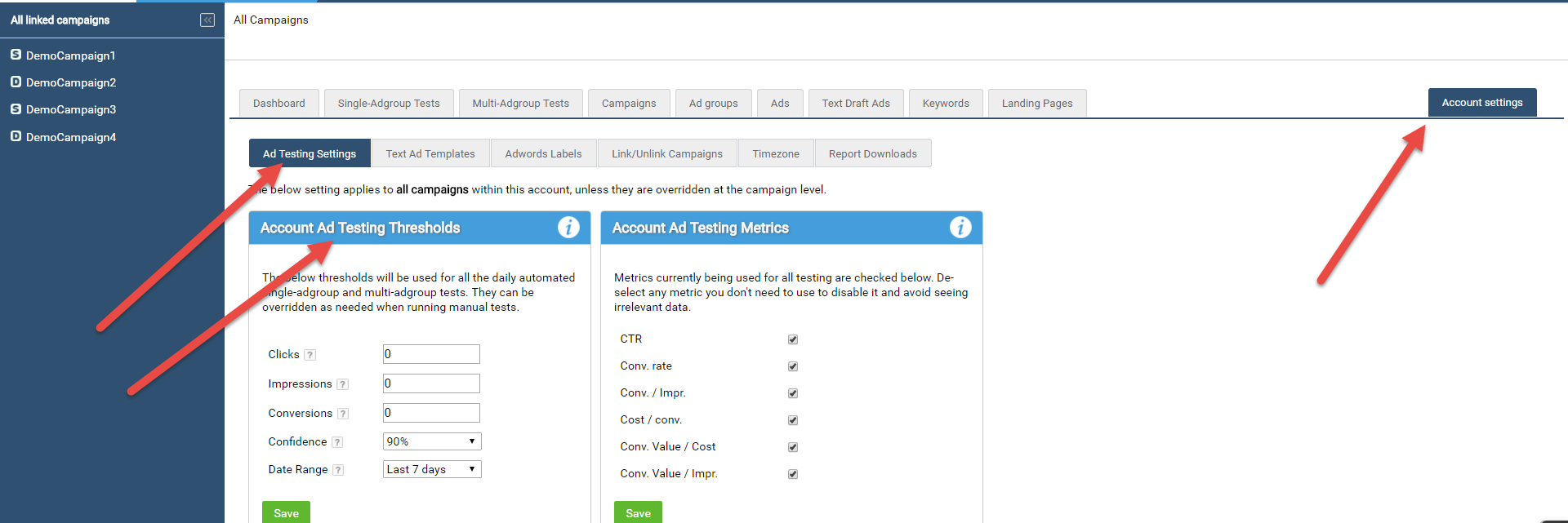In a single-adgroup test, a threshold defines the minimum performance each Ad must have, or a statistical confidence a test must achieve, before a valid test result is produced.

1. Daily automated single-adgroup tests: the thresholds are used for all test runs.  Account thresholds can be overridden at the campaign level.
2. Manual single-adgroup tests: you can override the defined thresholds for every manual test you run.

In a multi-adgroup test, a threshold is the minimum performance each set of Ads (defined by patterns or labels) must have, or a statistical confidence a test must achieve, before a valid test result is produced.

1. Daily automated multi-adgroup tests: the thresholds are used for all test runs.  Account thresholds can be overridden at the campaign level.
2. Manual multi-adgroup tests: you can override the defined thresholds for every manual test you run (as shown above).

How Thresholds affect the Metrics being Tested

A threshold is used only when it is relevant to the metric being calculated:

• Clicks threshold: used when calculating the CTR and Conv. Rate metrics only.
• Impressions threshold: used when calculating the CTR, Conv. /Impr. and Conv. Value / Impr. metrics only.
• Conversions threshold: used when calculating all metrics, except CTR.
• Confidence and Date Range thresholds: used when calculating all metrics.

• ### How Much Data Should You Have Before Examining an Ad Test Result?

Where are the Thresholds Defined?4. Related Info